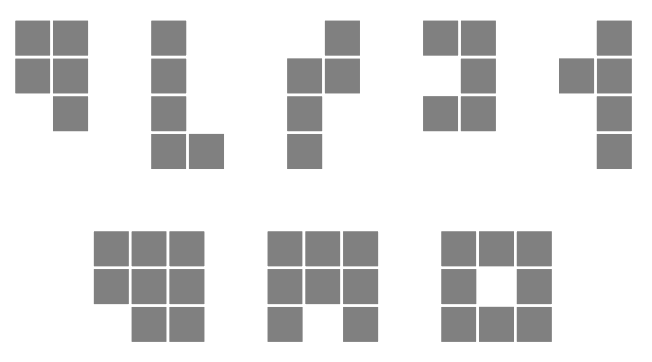시간 제한 메모리 제한 제출 정답 맞은 사람 정답 비율
2 초 128 MB 0 0 0 0.000%

## 문제

Lattice animal is a set of connected sites on a lattice. Lattice animals on a square lattice are especially popular subject of study and are also known as polyominoes. Polyomino is usually represented as a set of sidewise connected squares. Polyomino with $n$ squares is called $n$-polyomino.

In this problem you are to find a number of distinct free $n$-polyominoes that fit into rectangle $w \times h$. Free polyominoes can be rotated and flipped over, so that their rotations and mirror images are considered to be the same.

For example, there are 5 different pentominoes (5-polyominoes) that fit into $2 \times 4$ rectangle and 3 different octominoes (8-polyominoes) that fit into $3 \times 3$ rectangle.## 입력

The input file consists of a single line with 3 integer numbers $n$, $w$, and $h$ ($1 \le n \le 10$, $1 \le w, h \le n$).

## 출력

Write to the output file a single integer number --- the number of distinct free $n$-polyominoes that fit into rectangle $w \times h$.

## 예제 입력 1

5 1 4


## 예제 출력 1

0


## 예제 입력 2

5 2 4


## 예제 출력 2

5


## 예제 입력 3

5 3 4


## 예제 출력 3

11


## 예제 입력 4

5 5 5


## 예제 출력 4

12


## 예제 입력 5

8 3 3


## 예제 출력 5

3